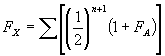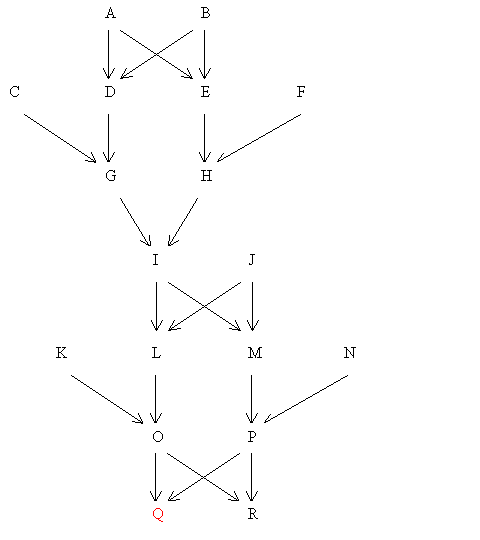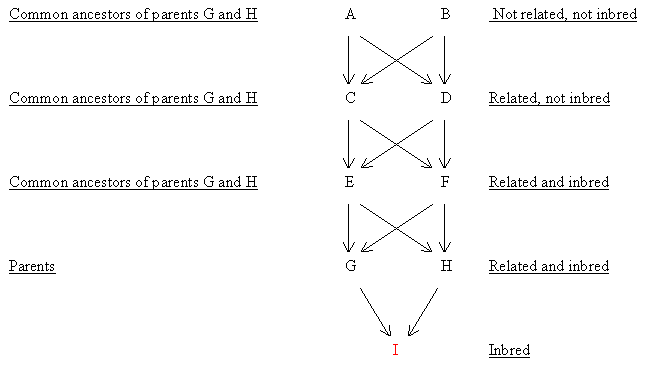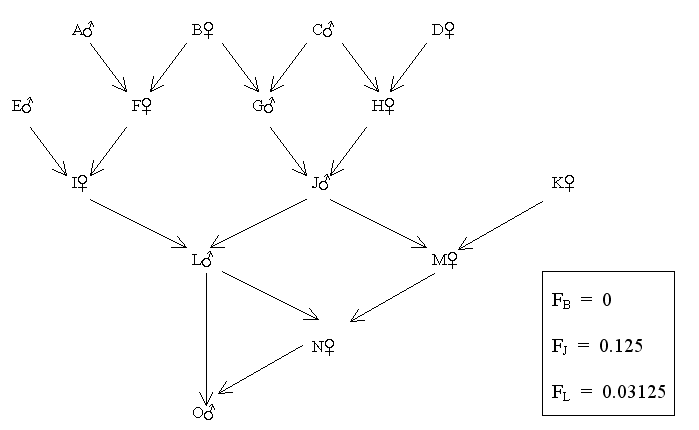THE COEFFICIENT OF INBREEDING (F) AND ITS APPLICATIONS

F measures the probability that two genes at any locus in an individual are identical by descent from the common ancestor(s) of the two parents. This means the degree to which two alleles are more likely to be homozygous (AA or aa) rather than heterozygous (Aa) in an individual, because the parents are related. Like R,F is a relative measure, in that there will be a certain level of homozygosity within the base population; F simply estimates the increase from that initial level as a result of recent inbreeding.

The inbreeding coefficient of an individual is approximately half the relationship (R) between the two parents.This equivalence only applies to low levels of inbreeding in an otherwise outbred population.e.g.Two single first cousins normally have a relationship (R) of 1/8.If there has been no previous inbreeding, their children will have a coefficient of inbreeding of 1/16.With high levels of continuous inbreeding this relationship breaks down. e.g.Some strains of laboratory rats and mice have reached an F value of 1.0, resulting from a long history of close inbreeding; but the coefficient of relationship (R) between any two members of the strain can never exceed 1.0. The mathematical reason for this is that although the basic formulae for R and F are Σ(1/2)n and Σ(1/2)n+1 respectively, as inbreeding within a line progresses, the correction terms applied to R for inbreeding (see here) gradually become more important and start to reduce the value of R below Σ(1/2)n. As F approaches 1.0, the correction terms for R also approach a maximum of (x 1/2); so that when F reaches 1.0 (complete homozygosity), R also becomes 1.0 and all members of the inbred line are identical.

The method of calculating the F coefficient of an individual is similar to that for the coefficient of relationship (R) between two collateral relatives, and involves the tracing of paths between the two parents via a common ancestor.The formula is as follows:-

Equation 1 method of calculating the F coefficient of an individualWhere FX is the coefficient of inbreeding of individual X, n is the number of connecting links between the two parents of X through common ancestors and FA is the coefficient of inbreeding of the common ancestor A.Thus, if the common ancestor is inbred, a minor calculation must be performed first to determine FA, before the main calculation can take place.In the main calculation any coefficients of paths through inbred common ancestors can then be multiplied by (1 + FA).Find FQ

The only inbred common ancestor of the two parents (O and P) is I (his parents are single first cousins).

a) First Find FI

 Common Ancestors (of parents G and H) Paths (1/2)n+1 (1 + FA) A G_1_D_2_A_3_E_4_H 1/32 x 1.0 = 1/32 B G_1_D_2_B_3_E_4_H 1/32 x 1.0 = 1/32 Therefore FI = Σ [(1/2)n+1(1 + FA)] = 1/16

i.e. FI = 0.0625 = 6.25%

b)      Main Calculation (To find FQ)

 Common Ancestors (of parents O and P) Paths (1/2)n+1 (1 + FA) I O_1_L_2_I_3_M_4_P 0.03125 x 1.0625 = 0.03220 J O_1_L_2_J_3_M_4_P 0.03125 x 1.0 = 0.03125 Therefore FQ = Σ[(1/2)n+1 (1 + FA)] = 0.06445

i.e. FQ = 0.06445 = 6.45%

Figure 65 Example without Inbred Common AncestorsFind FO

None of the common ancestors A, B, G or H are inbred. Therefore, no corrections are necessary.

 Common Ancestors (of parents M and N) Paths (1/2)n+1 G M_1_J_2_G_3_K_4_N 1/32 = 0.03125 H M_1_J_2_H_3_K_4_N 1/32 = 0.03125 A M_1_J_2_G_3_D_4_A_5_E_6_H_7_K_8_N M_1_J_2_H_3_E_4_A_5_D_6_G_7_K_8_N 1/512 x 4 = 0.0078125 B M_1_J_2_G_3_D_4_B_5_E_6_H_7_K_8_N M_1_J_2_H_3_E_4_B_5_D_6_G_7_K_8_N Therefore FO = Σ(1/2)n+1 = .0703125

i.e. FO = 7.03%

Figure 66 Close Inbreeding as used for Animals (3 generations of full sib mating)Find FI

Common ancestors of G and H are A, B, C, D, E and F.The only two that are inbred are E and F (related parents).Therefore, first calculate FE and FF (the same).

a)      Find FE and FF

 Common ancestors (of parents C and D) Paths (1/2)n+1 A C_1_A_2_D 1/8 Therefore, B C_1_B_2_D 1/8 FE = FF = 1/4 = 25%

b) Main Calculation (To Find FI)

 Common ancestors (of parents G and H) Paths (1/2)n+1 (1 + FA) E G_1_E_2_H (1/2)3 x 1.25 = 0.15625 F G_1_F_2_H (1/2)3 x 1.25 = 0.15625 C G_1_E_2_C_3_F_4_H (1/2)5 x 1.0 = 0.03125 G_1_F_2_C_3_E_4_H (1/2)5 x 1.0 = 0.03125 D G_1_E_2_D_3_F_4_H (1/2)5 x 1.0 = 0.03125 G_1_F_2_D_3_E_4_H (1/2)5 x 1.0 = 0.03125 A G_1_E_2_C_3_A_4_D_5_F_6_H (1/2)7 x 8 * 1.0 = 0.06250 G_1_F_2_C_3_A_4_D_5_E_6_H G_1_E_2_D_3_A_4_C_5_F_6_H G_1_F_2_D_3_A_4_C_5_E_6_H B G_1_E_2_C_3_B_4_D_5_F_6_H G_1_F_2_C_3_B_4_D_5_E_6_H G_1_E_2_D_3_B_4_C_5_F_6_H G_1_F_2_D_3_B_4_C_5_E_6_H FI=Σ[(1/2)n+1 (1 + FA)] = 0.50000

i.e FI =50%

The Closest Form of Inbreeding

The closest form of inbreeding is self-fertilisation which normally only occurs in monoecious plants and animals which are hermaphrodites,, e.g. garden peas and slugs.The equivalent situation has been experimentally produced in turkeys where a rare form of parthenogenesis occurs.Parthenogenesis (virgin birth) is the production of viable embryos (always males in birds) from haploid infertile eggs by the artificial doubling of chromosome numbers. The embryos are highly homozygous.If one of these parthenogenetic males is mated back to his mother this is equivalent to self fertilisation. (Olsen, 1965)

One generation of self-fertilisation produces the same coefficient of inbreeding (F) as three generations of full sib mating, i.e. 0.5.This follows from the formula for F, which is Σ(1/2)n+1 where n is the number of connecting links between the two parents via a common ancestor. With selfing, both parents are the same individual so that the number of links (n) is 0 and, therefore Σ(1/2)n+1 = 0.5.

Sib Marriages in Humans

In some societies close consanguineous marriages have been encouraged.For example, the ancient Egyptians and the Incas favoured marriages of brothers and sisters of the reigning dynasty.Inbreeding Coefficients F a) Amenhotep I and Aahotep II 25% b) Aames 37.5% c) Hatsheput 25% d) The rest of the individuals in the pedigree are not inbred, i.e. F = 0.

Double Grandchildren - The offspring of a full sib mating are sometimes referred to as double grandchildren because they only have two grandparents instead of the usual four (see here).

The Special Case of Directly Related Parents

In an incestuous situation where there is a close direct relationship between the two parents, such as father-daughter, mother-son or grandparent-grandchild, they may have no common ancestors in any previous generation, even though they have a strong genetic link. This is because one parent is the direct ancestor of the other. In these situations, despite having no common ancestors, the correction term (1 + FA) must still be applied to the path coefficient between an inbred ancestor (A) and his/her descendant partner.

 Figure 68 Father-daughter MatingThe reason is that, taking a father-daughter mating as an example; if the father (A) is inbred, his extra homozygosity will make it more likely that he will transmit to his grandson (D) further copies of the same alleles which his daughter (C) has already received from him. Since about one half of the genes the daughter receives from her father will also be passed on to the grandson, the latter's inbreeding coefficient (F) will rise above the normally expected level (0.25) and its value should be adjusted accordingly. (2 examples).Find FH                       FE = 0.25

 Common ancestors(of parents E and G Path (1/2)n+1 (1 + FA) * None E - G (1/2)2 x 1.25 = 0.3125 Therefore, FH= Σ[(1/2)n+1(1 + FA)] = 31.3%

However, there are cases (usually only found in animal breeding) where directly related parents do share common ancestors. If any of these common ancestors are also inbred, the correction term

(1 + FA) will be required in both situations.Find FO

 Common ancestors(of parents L and N) Paths (1/2)n+1 (1 + FA) * None L - N (1/2)2 x 1.03125 = 0.2578125 J L - J - M - N (1/2)4 x 1.125 = 0.0703125 B L - I - F - B - G - J - M - N (1/2)8 x 1.0 = 0.00390625 0.33203125 Therefore FO = ∑[(1/2)n+1(1 + FA)] = 33.2%

_________________________________________________________________________________

* A refers to any relevant inbred direct ancestor or common ancestor.

__________________________________________________________________________________

An alternative method of computing F is to use the technique of 'Coancestries' (Falconer, 1989).Instead of working from the present back to common ancestors we work forward, keeping a running tally, generation by generation, and compute the inbreeding that will result from the matings now being made.This method is easier than path coefficients for animal breeding programmes where the paths are often numerous and complex but unnecessary for normal human pedigrees.

For regular systems of inbreeding, as used in the 'inbred-hybrid' system for breeding chickens and maize, 'recurrence equations' are the only easy method for calculating F. A regular system of inbreeding is where a certain type of mating such as brother-sister, is repeated indefinitely (see Figure 66). A recurrence equation calculates the F value of the present generation from those of recent previous ones. e.g. the recurrence equation for repeated full sib mating is:

Ft = 0.25 (1 + 2Ft-1+ Ft-2) (Falconer, 1989)

Where Ft is the coefficient of the present generation, Ft-1 is the coefficient of the previous generation and Ft-2 is the coefficient of the generation before that. It is important to note that recurrence equations can only be used for regular systems of inbreeding. e.g. Three generations of full sib mating:-

 First generation - F1 = 0.25 (1 + 0 + 0) = 0.25 Second generation - F2 = 0.25 (1 + 0.5 + 0) = 0.375 Third generation - F3 = 0.25 (1 + 0.75 + 0.25) = 0.5

Table 19 Values of F for Consanguinous Matings (One generation, no previous inbreeding).

 Self fertilisation 1/2 Full sibs, Parent-child, Double first cousins (first degree) 1/4 Half sibs, Grandparent-grandchild, Uncle-niece, Double first cousins 1/8 First cousins 1/16 First cousins (once removed) 1/32 Second cousins 1/64 Second cousins (once removed) 1/128 Third cousins 1/256

Practical Uses of F

F is a very valuable parameter in both population and quantitative genetics.From the genealogists point of view the following are perhaps the two most interesting applications:

a) Predicting the Effects of Inbreeding Depression

A decline in performance in certain economic characters in domestic animals is well known following inbreeding. A similar depression has also been observed in humans.e.g.

Table 20 Inbreeding Depression for Every 10% Increase in F

 Animal Characteristic Inbreeding Depression Reference Chickens Hatchability 4.36% Shoffner (1948) Annual egg production 9.26 eggs Shoffner (1948) Man Height at age 10 2.0 cm Falconer (1989) I.Q. score 4.4% Falconer (1989) Pigs Body weight (154 days) 2.6 kg Falconer (1989) Litter size 0.24 piglets Falconer (1989) Cattle Annual milk yield 135 kg Falconer (1989) Sheep Fleece weight (1 year) 0.29 kg Falconer (1989)

Thus, 3 generations of full sib matings, as shown here, would lead to an expected decrease in egg production in chickens of 9.26 x 5 = 46.3 i.e 46 eggs, compared with other hens from the same population who were not inbred.

b)      Assessing the Risk of Inheriting Genetic Defects

In a large random-mating population, where the frequency of a harmful recessive gene (a) is q, the proportions of affected individuals and 'carriers' can be estimated from the Hardy-Weinberg Law as follows:

 Aa (carriers) aa (affected) 2q(1 − q) q2

However, if any inbreeding has occurred , Wright's Equilibrium Law enables a further prediction to be made about the increased risk of inheriting any harmful conditions caused by homozygous recessive genes.The expected frequency following inbreeding rises to:

aa (affected)

q2 + Fq(1 - q)

The following table shows how inbreeding increases the likelihood of inheriting three harmful recessive conditions in humans: phenylketonuria, albinism and alkaptonuria.The first and last of these three are serious metabolic disorders.

 Conditions Caused by Homozygous Recessive Genes Frequency of Recessive Gene (a)q Random Mating Proportion of Affected(aa) Following Inbreeding q2 + Fq(1 - q)  Proportion of Carriers (Aa) 2q(1 - q)  Proportion of Affected (aa) q2 First Cousin Marriage (F = 1/16) Full Sib Mating (F = 1/4) Phenylketonuria 1/100 1/50 1/10,000 1/1,380 1/385 Albinism 1/141 1/70 1/20,000 1/2,000 1/550 Alkaptonuria 1/1,000 1/500 1/1,000,000 1/16,000 1/4,000

Therefore, with albinism for example, a first cousin marriage increases the risk of inheriting the condition ten-fold, and with alkaptonuria the increase following a full sib mating is 250 fold. It also comes as quite a shock to most people that, even without inbreeding, the proportion of normal people carrying phenylketonuria is as high as 1 in 50.

 Some of the proportions are rounded off and all are expressed as unit fractions for greater clarity

 The reason for this correction is that if a common ancestor of the two parents is inbred, he or she will have a greater degree of homozygosity than normal.This increases the probability that a descendant's parents will both receive the same gene from that common ancestor, which in turn will raise the level of homozygosity of the descendant over and above that normally determined by the relationship between the parents.

 When q is very small 2q(1-q) approximates to 2q, and Fq(1-q) to Fq